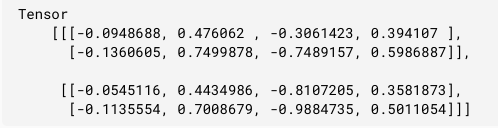GFG App
Open AppBrowser
Continue

# Tensorflow.js tf.layers.gru() Function

Tensorflow.js is an open-source library developed by Google for running machine learning models and deep learning neural networks in the browser or node environment.

Tensorflow.js tf.layers.gru() function is used to create a RNN layer which consists of only one GRUCell and the apply method of this layer operates on a sequence of input tensors. The shape of input tensor must be atleast 2D and the first dimension must be time steps. gru is Gated Recurrent Unit.

Syntax:

`tf.layers.gru(args)`

Parameters:

• args: It specifies the given config object.
1. recurrentActivation: It specifies the activation function which will be used for the recurrent step. The default value of this parameter is hard sigmoid.
2. implementation: It specifies the implementation mode. It can be either 1 or 2. For superior performance implementation is recommended.

Return value: It returns a tf.layers.Layer

Example 1:

## Javascript

 `// Importing the tensorflow.js library  ` `const tf = require(``"@tensorflow/tfjs"``);  ` ` `  `// Create a RNN model with gru Layer ` `const RNN = tf.layers.gru({units: 8, returnSequences: ``true``}); ` ` `  `// Create an input which will have 5 time steps ` `const input = tf.input({shape: [5, 10]}); ` `const output = RNN.apply(input); ` ` `  `console.log(JSON.stringify(output.shape));`

Output:

`[null, 5, 8]`

Example 2:

## Javascript

 `// Importing the tensorflow.js library  ` `const tf = require(``"@tensorflow/tfjs"``);  ` ` `  `// Create a new model with gru Layer ` `const rnn = tf.layers.gru({units: 4, returnSequences: ``true``}); ` ` `  `// Create a 3d tensor ` `const x = tf.tensor3d([ ` `    ``[ ` `        ``[1, 2], ` `        ``[3, 4], ` `    ``], ` `    ``[ ` `        ``[5, 6], ` `        ``[7, 8], ` `    ``], ` `]); ` ` `  `// Apply gru layer to x ` `const output = rnn.apply(x); ` ` `  `// Print output ` `output.print()`

Output:My Personal Notes arrow_drop_up